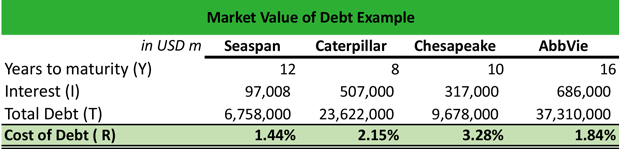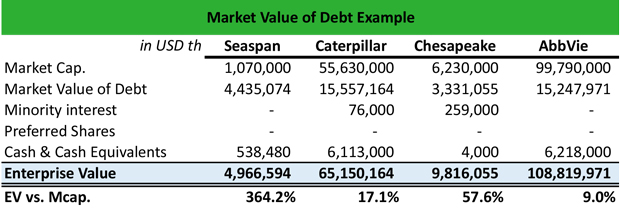# What is Market Value of Debt?

Definition: The market value of debt refers to the amount of bank debt that firms have but do not directly report on their balance sheet. Hence, it has to be calculated.

## What Does Market Value of Debt Mean?

What is the definition of market value of debt? Firms report the book value of debt on their financial statements and not their bank debt. Although the book value of debt is most commonly used in empirical finance, the market value of debt is more accurate because it involves both the cash and debt of a firm, thereby taking into account the firm’s capital structure. Also, the market value of debt helps analysts to calculate the enterprise value of a firm, which is higher than the market cap if the company carries a lot of debt.

The simplest way to estimate the market value of debt is to convert the book value of debt in market value of debt by assuming the total debt as a single coupon bond with a coupon equal to the value of interest expenses on the total debt and the maturity equal to the weighted average maturity of the debt. Therefore, if the market value of the debt is \$1,000,000, the interest expenses are \$60,000 and the maturity is 5 years, and the current cost of debt is 8%, then the market value of debt is: 60 x [1-(1/(1.08^5)) /0.08]+1000/(1.08^5) = 60 x [(1-0.6805) /0.086] + 1000/ 1.469 = 60 x (0.3194/ 0.08) + 680.58 = (60 x 3.9927) + 680.58 = 239.56 + 680.58 = 920.15.

Let’s look at an example.

## Example

Anna holds a portfolio of four stocks, which trade in different sectors. In order to properly evaluate the enterprise value of each company and determine the level of debt that each company carries, she is set to calculate the market value of debt by implementing the following process:

1. First, she calculates the cost of debt by dividing the interest over the total debt. Hence, for Seaspan, the cost of debt is \$97,0008/\$6,758,000 = 1.44%, for Caterpillar is \$507,000/\$23,622,000 = 2.15%, and so on.

2. Then, she assumes the years to maturity based on company information. Hence, for Seaspan is 12 years, for Caterpillar 8, for Chesapeake 10, and for Abbvie 16. These will be used in the calculation of the market value of debt.3. The next step is to calculate the market value of debt by employing the formula:

MV of debt = Interest x ((1-(1/1+Cost of debt) ^Years)) /Cost of debt + Total Debt/ (1+Cost of debt) ^Years Hence:

MV of Debt Seaspan: 97,008 x ((1-(1/1+1.44%) ^12)) / 1.44% + 6,758,000 / (1+1.44%) ^12 = 4,435,074

MV of Debt Caterpillar: 507,000 x ((1-(1/1+2.15%) ^8)) / 2.15% + 23,622,000 / (1+2.15%) ^8 = 15,557,164

MV of Debt Chesapeake: 317,000 x ((1-(1/1+3.28%) ^10)) / 3.28% + 9,678,000 / (1+3.28%) ^10 = 3,331,055

MV of Debt AbbVie: 686,000 x ((1-(1/1+1.84%) ^16)) / 1.84% +37,310,000 / (1+1.84%) ^16 = 15,247,971Notice that the enterprise value is higher than the market cap in all cases because it includes the debt of the company.

## Summary Definition

Define Market Value of Debt: MVD means off balance sheet obligations.